# Study of the Project Parameters Influence in the Performance of Solar Collectors

Study of the Project Parameters Influence in the Performance of Solar Collectors

Lidianne de P.P MapaBárbara de M. Mendes Luís A. Bortolaia Elisangela M. Leal

Graduate Program in Civil Engineering (PROPEC), Federal University of Ouro Preto, Ouro Preto, Minas Gerais, Brazil

Department of Mechanical Engineering (DEMEC), Federal University of Ouro Preto, School of Mining, Campus Morro do Cruzeiro, ZIP: 35400-000, Ouro Preto, Minas Gerais, Brazil

Corresponding Author Email:
lidianne.pinto@aluno.ufop.edu.br
Page:
313-321
|
DOI:
https://doi.org/10.18280/ijht.370137
24 October 2018
|
Accepted:
10 March 2019
|
Published:
31 March 2019
| Citation

OPEN ACCESS

Abstract:

This paper aims to observe the influence of design parameters on the performance of plane solar collectors. From the energy balance of the plane solar collector, the mathematical model was implemented varying the following design parameters: (i) Thickness of the absorber plate; (ii) Distance between the absorber and the cover; (iii) Edge insulation thickness; (iv) Absorber emittance; (v) Conductivity of the absorber; (vi) Convective heat transfer coefficient inside the tubes; (vii) Distance between tubes; (viii) Wind velocity; (ix) Solar radiation incident; and (x) Back insulation thickness. These parameters were altered observing the influence on the optical efficiency; the coefficient of energy loss; the instantaneous efficiency; and the useful energy gain. From the results, it is possible to see that the parameters that most influence the performance of the solar collector are the distance between the absorber and the cover, the absorber emittance, the thermal conductivity of the absorber, the distance between tubes, wind speed and solar radiation incident.

Keywords:

coefficient of energy loss, instantaneous efficiency, optical efficiency, plane solar collector, useful energy gain

1. Introduction

Solar energy is a renewable energy source, which enables the reduction of environmental impacts and investments in energy distribution and transmission. The Earth receives 173000 TW of solar radiation [1-2]. The atmosphere absorbs approximately 19% of this energy, 35 % is reflected by clouds and 46% is absorbed directly and indirectly by the Earth [3-4]. Solar radiation as a source of energy has several applications such as heating fluids and environments and generating mechanical or electrical power .

In Brazil, a high consumption of energy for heating water in homes is found. According to , electric showers are present in more than 90 % of Brazilian residences and account for about 60 % of the residential electricity load during peak hours, which is from 6 pm to 9 pm.

The energy use for heating fluids can be done through solar energy, allows the reduction of the use of other energy sources (e.g. electrical energy), has bigger environmental and social impacts, and benefits the system of energy generation and distribution .

Solar collectors heating systems permit the fluids heating by absorbing solar energy and transforming it into thermal energy . Plane solar collectors are the most commonly used in homes because of their low cost and ease of deployment . These are composed of three main items, as shown in Figure 1: (i) Solar energy absorbing surface, which is usually made of copper or aluminum and transfers the absorbed energy to the fluid contained in the riser tubes; (ii) Glass cover for the use in the wavelength range of the solar radiation incident on the absorbing surface and opaque in the emission region of the absorbing plate in order to reduce the losses due to the convection and radiation to the atmosphere; and (iii) Thermal insulation to reduce conduction losses [10-11].

## 1.png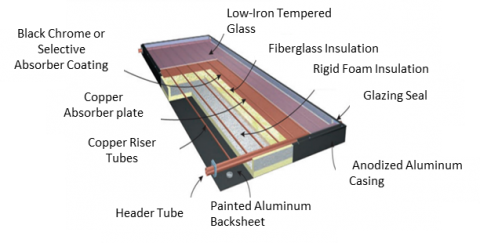Figure 1. Typical plane solar collector 

The energy balance in the complete collector considering steady-state conditions and tube and fin type construction is defined as :

[Gain of thermal energy in the collector]=[Energy absorbed by the plate and tubes]-[Heat loses to the ambient]

Thus, the equation of the useful energy gain (Qu) of a plane collector is related to the both absorbed solar radiation and thermal energy loss [3, 10].

Qu=At FR [GT (τα)-UL (Tm-Ta)] (1)

where, At is the total area of the collector (m²); FR is the heat removal factor of the collector; GT is the solar radiation incident (W/m2); t is the glass transmittance; α is the absorber plate absorptance; UL is the collector overall heat loss coefficient (W/m2.K); Tm is the average inside fluid temperature at the collector (K), represented by the average inlet fluid temperature (Ti) and the average outlet fluid temperature (To); Tthe ambient temperature (K).

The heat removal factor of the solar collector for parallel tubes is given by [13, 10]:

${{F}_{R}}=\frac{\dot{m}Cp}{{{A}_{t}}{{U}_{L}}}[1-\exp (-\frac{{F}'{{U}_{L}}{{A}_{t}}}{\dot{m}Cp})]$ (2)

where $\dot{m}$ is total mass flow of the working fluid (kg/s), F' is efficiency factor of the solar collector, Cp is the specific heat capacity of fluid (J/Kg K).

Góngora-Gallardo et al.  affirm that the efficiency factor of the solar collector (F') is related to the structural geometry of the collector. This factor is given by:

$F'=\frac{1/{{U}_{L}}}{W[\frac{1}{{{U}_{L}}|F(W-D)+D|}+\frac{1}{{{C}_{b}}}+\frac{1}{\pi D{{h}_{i}}}]}$ (3)

where hi is the convective heat transfer coefficient inside the tubes (W/m2.K), D is the outside diameter of the collecting tubes (m), F is the fin efficiency factor for straight fins with rectangular profile. It is considered that the region between the centerline separating the tubes and the tube base is a classical fin problem. The fin efficiency factor (F) can be calculated as :

$F=\frac{\tanh [{}^{m(W-D)}/{}_{2}]}{\left\lceil {}^{m(W-D)}/{}_{2} \right\rceil }$ (4)

$m=\sqrt{{{{U}_{L}}}/{{{K}_{abs}}\delta }\;}$ (5)

where, kabs is the thermal conductivity of the absorber material (W/m.K); δ is the thickness of the absorber (m), W is the distance between tubes (m), which can be calculated from :

$W=\frac{D+{{L}_{C}}}{NT+1}$ (6)

where, LC is the width of the collector (m); and NT is the number of collector tubes. The collector overall heat loss coefficient by the collector (UL) is given by :

UL=Ut+Ub+U(7)

where, Ut is the top heat loss coefficient (W/m² K); Ub is the bottom heat loss coefficient (W/m²K); Ue is the edge heat loss coefficient (W/m²K).

The collector top heat loss coefficient associates the radiative and convective heat transfer. The calculation of Ut can be written as :

${{U}_{L}}\text{=}{{\left\{ \frac{N}{\frac{204.48}{{{T}_{P}}}{{[{{L}^{3}}\cos \beta \frac{({{T}_{P}}-{{T}_{a}})}{N+f}]}^{0.252}}}+\frac{1}{{{h}_{W}}} \right\}}^{-1}}+\left\{ \frac{\sigma ({{T}_{P}}-{{T}_{a}})(T_{P}^{2}+T_{a}^{2})}{{{({{\varepsilon }_{P}}+0.0425(1-{{\varepsilon }_{P}}))}^{-1}}+[\frac{(2N+f-1)}{{{\varepsilon }_{g}}}]-N} \right\}$ (8)

where, N is the number of glass covers; L is the distance between the absorber and the cover (m); β is the inclination of the solar collector (°); hw is the convective heat transfer coefficient due to wind (W/m².K), and f is a factor, which can be calculated as :

$f=(\frac{9}{{{h}_{W}}}-\frac{30}{h_{W}^{2}})(\frac{{{T}_{a}}}{316.9})(1+0.091N)$ (9)

Heat loss by convection between the cover and the environment is due to the wind action . The determination of the convective heat transfer coefficient (hw) between the cover and the ambient air is performed through equation :

hw=5,7+3,8Vw ,                      Vw≤5m/s (10)

where, Vw is the average wind speed (m/s).

The bottom and edge heat loss of the collector results mainly from the heat transfer resistance by conduction throughout insulation. The bottom heat loss coefficient (Ub) can be expressed by [17-18]:

${{U}_{b}}=\frac{k}{{{\delta }_{2}}}$ (11)

where, k the thermal conductivity of the insulation (W/m.K); and δ2 the back insulation thickness (m).

The edge heat loss coefficient (Ue) is determined from :

${{U}_{e}}=\frac{\frac{k}{{{\delta }_{1}}}{{P}_{c}}{{\delta }_{c}}}{{{A}_{t}}}$ (12)

where, δ1 is the edge insulation thickness (m); Pc the collector perimeter (m); δc the thickness of the collector (m).

The optical efficiency (h0) of the plane collectors is determined from the transmittance of the cover (τ) and the absorptance (α) of the absorber plate as :

η0=τα (13)

The instantaneous efficiency (hi) of the solar collector can be determined by the ratio of the useful energy gain (Qu) to the incident solar radiation. The equation representing the Instantaneous efficiency is expressed by [19-20]:

${{\eta }_{i}}=\frac{{{Q}_{u}}}{{{A}_{t}}{{G}_{T}}}$ (14)

Substituting the useful energy gain in equation (14), one can obtain :

${{\eta }_{i}}=\frac{{{Q}_{u}}}{{{A}_{t}}{{G}_{T}}}=\frac{{{A}_{t}}{{F}_{R}}[{{G}_{T}}(\tau \alpha )-{{U}_{L}}({{T}_{m}}-{{T}_{a}})]}{{{A}_{t}}{{G}_{T}}}={{F}_{R}}(\tau \alpha )-{{F}_{R}}{{U}_{L}}\frac{({{T}_{m}}-{{T}_{a}})}{{{G}_{T}}}$ (15)

In equation (15), the parameter [FR (τα)] (optical efficiency) indicates how energy is absorbed and the parameter ${{F}_{R}}{{U}_{L}}\frac{({{T}_{m}}-{{T}_{a}})}{{{G}_{T}}}$  indicates how energy is lost. In this work, the parameter ${{F}_{R}}{{U}_{L}}\frac{({{T}_{m}}-{{T}_{a}})}{{{G}_{T}}}$ will be designated as the coefficient of energy loss.

The performance of plane solar collectors is related to the project parameters and to the climate factors . Therefore, this work contribution is to analyze the performance factors of plane solar collectors, such as the optical efficiency, the coefficient of energy loss, the instantaneous efficiency and the useful energy gain, with the variation of the parameters: (i) Thickness of the absorber plate; (ii) Distance between the absorber and the cover; (iii) Edge insulation thickness; (iv) Absorber emittance; (v) Conductivity of the absorber; (vi) Convective heat transfer coefficient inside the tubes; (vii) Distance between tubes; (viii) Wind velocity; (ix) Solar radiation incident; (x) Back insulation thickness.

2. Materials and Methods

From the application of the energy balance in the collector, the mathematical model was implemented for the determination of optical efficiency, coefficient of energy loss, instantaneous efficiency and useful energy gain of the collector.

The calculations were accomplished using typical data of the local environment and components of the solar collector. From the initial values, variations in the parameters such as thickness of the absorber plate, distance between the absorber and the cover, edge insulation thickness, absorber emittance, absorber conductivity, convective heat transfer coefficient inside the tubes, distance between tubes, wind speed, solar radiation incident and back insulation were made.

The initial data of ambient temperature (20 ºC), average temperature of the absorber plate (80 ºC), inlet water temperature (25 ºC), outlet water temperature (50 ºC); wind speed (3 m/s) and solar radiation incidence (1000 W/m²) were used. In addition to the initial data, the constants of Stefan-Boltzmann of 5,67×10-8 W/m2K4 and number of covers of 1, were used.

Table 1. Materials properties and other collector variables 

 Variable Collector Glass emittance (εg) 0.88 Absorber plate emittance (εp) 0.96 Glass transmittance (τ) 0.885 Absorptance (α) 0.95 Collector inclination (β) 45° Convective heat transfer coefficient (hi) 300 W/m²K Bond conductance (Cb) 95 W/m.K Insulation conductivity – KRockwool 0.045 W/m.K Absorber plate conductivity – KCopper 380 W/m.K Specific heat at constant pressure of the of the working fluid (average) 4180 J/kg.K

Table 2. Collector dimensions [10, 22]

 Variable Collector Collector thickness (δc) 0.085 m Collector width (Lc) 1.148 m Collector length (Cc) 2.003 m Collector total area (At) 2.30 m2 Collector perimeter (Pc) 6.302 m Back insulation thickness (δ2) 0.04 m Edge insulation thickness (δ1) 0.02 m Thickness of the glass cover (Lg) 0.0032m Thickness of the absorber (δ) 0.0005 m Distance between the absorber and the cover (L) 0.022 m Outside tube diameter (D) 0.02 m Distance between tubes (W) 0.090 m Number of tubes (NT) 12

Table 1 shows the materials properties data, the inclination of the collector and the convective heat transfer coefficient inside the tubes used in the calculation.

The collector dimensions used for the calculation are shown in Table 2.

Figure 2 and Figure 3 show respectively the top and cross-sectional views of a plane solar collector with the dimensions used for the calculation.

## 2.png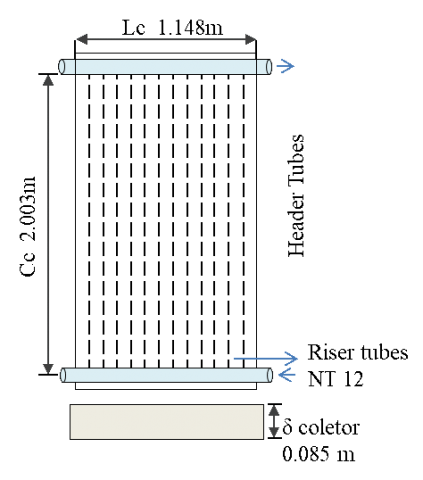Figure 2. Top view of a plane solar collector [10, 22]

## 3.pngFigure 3. Cross section of a plane solar collectorigure 2 and Figure 3 show respectively the top and cross-sectional views of a plane solar collector with the dimensions used for the calculation

3. Results and Discussion

3.1 Heat losses, efficiency and useful energy gain

Throughout the initial data (Table 1 and Table 2), it was possible to calculate the main losses in the collector, the optical and instantaneous efficiency, as shown in Table 3.

Table 3. Results of the main losses and efficiencies of the plane collector

 Variable Collector Useful energy gain (Qu) 1474.14 W wWW Collector efficiency factor (F') 0.94 Overall heat loss coefficient (UL) 8.99 W/m²K Top heat loss coefficient (Ut) 7.34 W/m²K Bottom heat loss coefficient (Ub) 1.12 W/m²K Edge heat loss coefficient (Ue) 0.52 W/m²K Optical efficiency (FR η0) 0.80 Coefficient of energy loss (FRUL ((Tm –Ta)/G)) 0.15 Instantaneous efficiency (ηi) 0.64

Table 3 shows the results obtained as a function of the initial data, without any variation of the parameters. According to Duffie and Beckman , the collector efficiency factor should not be less than 0.9, in order to have a good solar collector efficiency. In this Table, it is observed that the collector efficiency is equal to 0.64 and the available heat to warm up the working fluid (water) is 1474.1 W.

3.2 Variation of the thickness of the absorber plate

The thickness of the absorber plate was varied from 0.4 to 1.5 mm. The result is shown in Figure 4.

## 4.png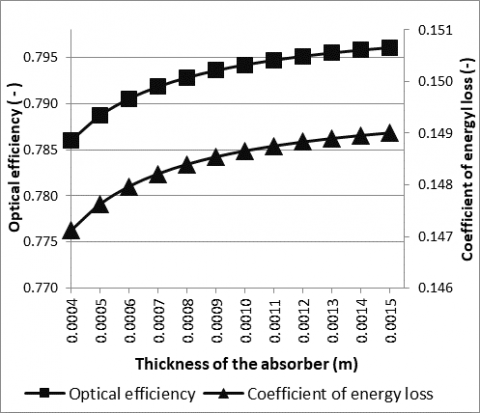Figure 4. Influence of the thickness of the absorber plate on the optical efficiency and the coefficient of energy loss

It can be observed that the higher the thickness, the greater the optical efficiency and coefficient of energy loss. The increase of the parameters occurs up to a thickness of approximately 0.13 mm. From this value, the parameters remain approximately constant. There is an increase of 1.27 % for the optical efficiency and an increase of 1.36 % of the coefficient of energy loss in the range of 0.4 to 1.5 mm of the thickness, due to the reduction of the conduction resistance between the absorber and the collector tubes.

## 5.png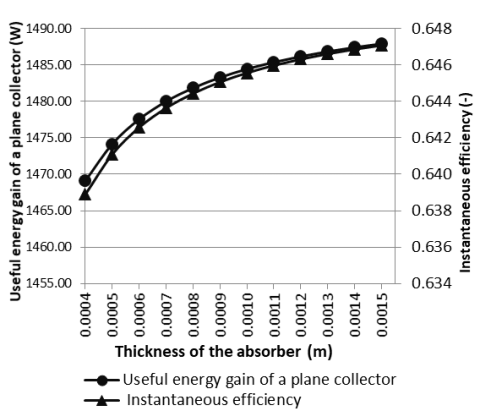Figure 5. Influence of the thickness of the absorber plate on the useful energy gain and the instantaneous efficiency

Figure 5 shows the influence of the thickness of the absorber plate on the instantaneous efficiency and useful energy gain by the collector. It is observed with the increase of the thickness of the absorber plate, the instantaneous efficiency increases by 1.25 % and the useful energy gain by 1.28 %.

3.3 Variation of the distance between the absorber plate and the cover

The distance between the absorber plate and the cover was varied from 5 mm to 39 mm for the adopted collector. The results are shown in Figure 6.

## 6.png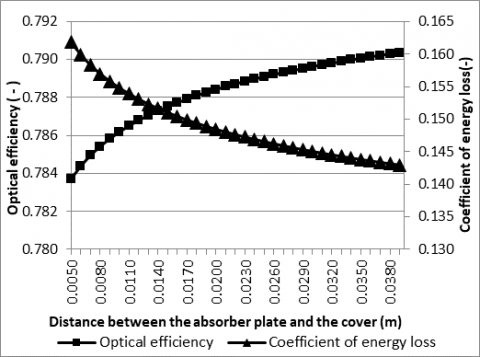Figure 6. Influence of the distance between the absorber plate and the coverage on the optical efficiency and the coefficient of energy loss

From Figure 6, it is observed that when the distance is increased, the coefficient of energy loss decreases and the optical efficiency increases. This is because of the reduction of the of the top heat loss coefficient. In overall, the coefficient of energy loss decreases by 11.73 % and the optical efficiency increases by 0.77 % in the range of the specified plate-to-cover distance.

Figure 7 shows the influence of the distance between the absorber and the cover in the instantaneous efficiency and the useful energy gain.

## 7.pngFigure 7. Influence of the absorber plate thickness on the useful energy and the instantaneous efficiency

The useful energy gain of a plane collector presents a variation of 4.10 % (from 1430 W to 1488.7 W) and a variation of the instantaneous efficiency of 4.02 % (from 0.622 to 0.647), showing that the variation of the distance between the absorber plate and the cover has a significant influence efficiency of the solar collector.

3.4 Variation of the edge insulation thickness

Data between 15 mm and 35 mm were used for edge insulation thickness. It can be seen in Figure 8 that the optical efficiency value increases by 0.25 % with the increase of the edge insulation thickness, but the coefficient of energy loss decreases by 4 %. This happens because the value of the collector overall heat loss coefficient decreases and the collector efficiency factor increases a bit, due to the increased resistance of the heat conduction to the ambient.

## 8.pngFigure 8. Influence of the edge insulation thickness on the optical efficiency and the coefficient of energy loss

Figure 9 shows the influence of the edge insulation thickness on the useful energy gain and the instantaneous efficiency.

Figure 9 indicates that the increase of the edge insulation from 15 mm to 35 mm rises the useful energy gain about 1.30 % and instantaneous efficiency about 1.40 %.

## 9.png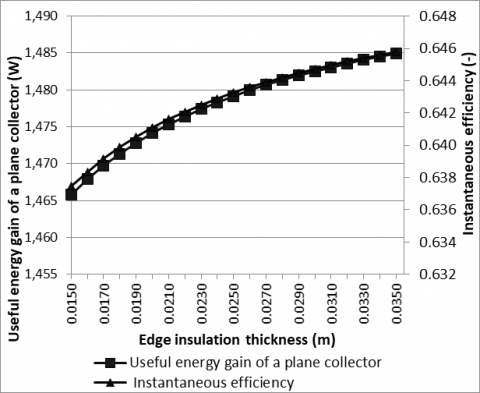Figure 9. Influence of the edge thickness on the useful energy and the instantaneous efficiency

3.5 Variation of the absorber emittance

The absorber emittance was varied from 0.05 to 0.96 and its influence on the optical efficiency and the coefficient of energy loss is shown in Figure 10. As can be seen in Figure 10, lower emittances have higher optical efficiencies and lower coefficients of energy loss. For being a parameter that greatly influences the collector, it is much studied and responsible for the improvements of solar collectors. The optical efficiency decreased by 2.71 % and the coefficient of heat loss increased by 74.12 %.

## 10.pngFigure 10. Influence of the emittance of the absorber on the optical efficiency and the coefficient of energy loss

Figure 11 displays the influence of the emittance of the absorber on the useful energy and the instantaneous efficiency. It can be seen that the useful energy gain and the instantaneous efficiency increase with the decrease of the emittance of the absorber plate.

## 11.pngFigure 11. Influence of the emittance of the absorber on the useful energy and the instantaneous efficiency

The instantaneous efficiency and the useful energy gain decrease of about 11.7 %, ranging from 0.726 to 0.641 and from 1670.4 W to 1474.1 W, respectively, with increasing emittance.

3.6 Variation of the absorber plate thermal conductivity

The absorber conductivity is ability to transfer heat to the working fluid; therefore, materials with higher conductivities allow greater heat transfer. Figure 12 and Figure 13 show the influence of the absorber conductivity variation from 50 to 400 W/(m.K) on the optical efficiency and the coefficient of energy loss and on the useful energy and instantaneous efficiency, respectively. It can be seen that the increase of the absorber conductivity rises the optical efficiency, the coefficient of energy loss; the useful energy gain as well as instantaneous efficiency. From 300 W/(m.K), there are a slight variation (asymptotic behavior) in the mentioned parameters.

In Figures 12 and 13, one can observe that the behavior of the curves with the increase of the thermal conductivity of the absorber plate. The variations obtained in the optical efficiency was of 8.68 %; in the coefficient of energy loss of 8.82 %; in the instantaneous efficiency of 8.81 %, and in the useful energy gain of 8.73 %.

## 12.pngFigure 12. Influence of absorber conductivity on the optical efficiency and in the coefficient of energy loss

## 13.pngFigure 13. Influence of absorber conductivity on the useful energy and the instantaneous efficiency

3.7 Variation of the convective heat transfer coefficient inside the tubes

The convective heat transfer coefficient inside the tubes was varied from 300 to 1000 W/(m²K). Figure 14 shows this variation on the optical efficiency and the coefficient of energy loss. It can be seen that as the convective coefficient increases, the optical efficiency of the collector increases of about 2.92 % because more heat is transferred to the working fluid. In addition, the increase of the convective heat transfer also rises the coefficient of energy loss of about 2.70 %.

## 14.pngFigure 14. Influence of the heat transfer coefficient inside the tubes on the optical efficiency and the coefficient of energy loss

## 15.png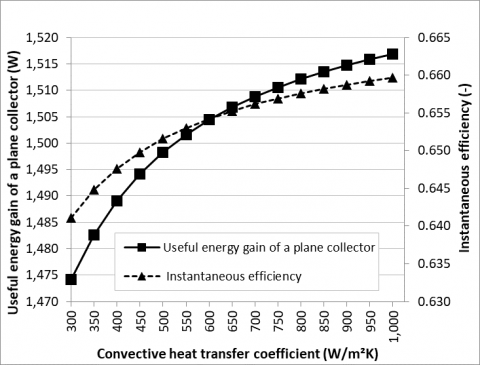Figure 15. Influence of the convective heat transfer coefficient inside the tubes in the useful energy gain and the instantaneous efficiency

Figure 15 shows the influence of the convective heat transfer coefficient inside the tubes in the useful energy gain and in the instantaneous efficiency, which presents the same behavior found in Figure 14. The increase in the convective heat transfer coefficient rises in 2.90 % the useful energy gain and the instantaneous efficiency.

3.8. Variation of the distance between tubes

The variation of the distance between tubes was studied and is shown in Figures 16 and 17. The distance was varied from 40 to 200 mm and the other parameters were kept constant. The increase in the distance between tubes decreases the number of tubes, the coefficient of energy loss (by 16.88 %), the optical efficiency (by 16.55 %), the instantaneous efficiency (by 16.50 %) and the useful energy gain (by 16.45 %). This result is due to the reduction of the heat transfer area to the working fluid due to the reduction in the number of tubes.

## 16.png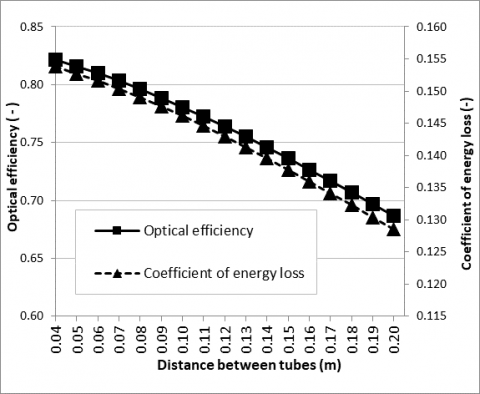Figure 16. Influence of distance between tubes on the optical efficiency and the coefficient of energy loss

## 17.png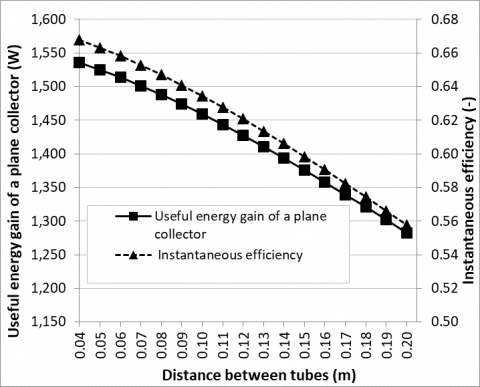Figure 17. Influence of the distance between tubes on the useful energy gain and the instantaneous efficiency

3.9 Variation of the wind speed

In the Figure 18, the wind speed was varied from 0 to 8.5 m/s. It directly influences the wind convective heat transfer coefficient and, therefore, the coefficient of energy loss of the collector, and the performance parameters analyzed. According to Figure 18, the optical efficiency presents a decrease of 1.63 %. On the other hand, the coefficient of energy loss increases strongly (around 29.9 %) with the increase of the wind speed. This happens because the coefficient of energy loss from the top of the collector has a greater influence on the absorbed solar energy, since the collector cover has no heating.

Figure 19 shows the influence of wind speed variation on the useful energy and the instantaneous efficiency. In this figure, one can observe that the instantaneous efficiency decreases in the order of 7.80 % on the increase in wind speed from 0.0 to 8.5 m/s. The influence of wind speed on the useful energy presents the same behavior, which represents a decrease of about 7.70 %.

## 18.png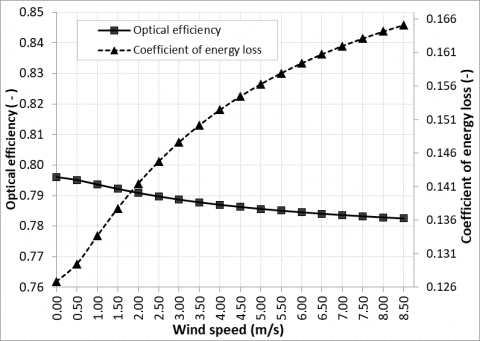Figure 18. Influence of wind speed on optical efficiency and coefficient of energy loss

## 19.pngFigure 19. Influence of wind speed on useful energy and instantaneous efficiency

3.10 Variation of the solar radiation incident and the back insulation

The influence of the solar radiation incident on optical efficiency, instantaneous efficiency, useful energy gain, and coefficient of energy loss was studied, and the results is presented in Figure 20. This parameter was varied from 500 to 1100 W/m² and the other parameters were kept constant. It is observed in Figure 20 that the increase in solar radiation does not influence the optical efficiency but decreases in 54.60% the coefficient of energy loss. In addition, is possible to observe that with the increase of the solar radiation incident, the useful energy has a gain of 191.80 % and the instantaneous efficiency has a gain of 32.80 %, confirming that the more availability of the solar radiation, the better the collector performance.

The influence of the back insulation thickness on the optical efficiency and the coefficient of energy loss was analyzed, varying the thickness of 20 to 50 mm. The results are shown in Figure 21. The increase of the back insulation thickness decreases the heat losses from the collector bottom to the ambient. Therefore, there is a decrease of the coefficient of energy loss by 12.73 % and a slight increase of 0.89 % in the optical efficiency.

## 20.pngFigure 20. Influence of solar radiation incident on optical efficiency, instantaneous efficiency, useful energy gain and coefficient of energy loss

## 21.png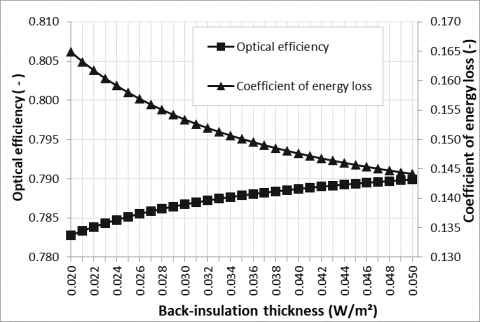Figure 21. Influence of back insulation thickness on optical efficiency and coefficient of energy loss

## 22.pngFigure 22. Influence of the back insulation thickness on useful energy gain and instantaneous efficiency

In Figure 22 is possible to observe that with the increase of the back insulation thickness, the useful energy gain and the instantaneous efficiency has a gain of approximately 4.50 % and is therefore significant.he thickness of the absorber plate was varied from 0.4 to 1.5 mm. The result is shown in Figure 4.

4. Conclusions

The main objective of this paper was to analyze the influence of design parameters on the performance of solar plane collectors for residential water heating. To perform a parametric study of this influence, the mathematical model of the plane solar collector was implemented.

As shown by the results obtained, the parameters, which most influence the performance of the solar collector, are the distance between the absorber and the cover, the emittance of the absorber, the thermal conductivity of the absorber, the distance between tubes, wind speed and solar radiation incident.

The distance between the absorber plate and the cover has a significant influence on the performance of the solar collector, and should be well dimensioned, as it allows a variation of 4.10 % in useful energy and 4.02 % in the efficiency instantaneous.

The emittance of the absorber plate is another parameter of great influence. With the increase of the emittance, in the proposed range of variation, the instantaneous efficiency and the useful energy gain decrease of approximately 11.70 %. For low emittance, the optical efficiency, which also depends on the transmittance of the cover, the useful energy gain and the instantaneous efficiency have higher values. For higher values of emittance, the coefficient of loss is higher, decreasing the values of the performance parameters.

Since the conductivity of the absorber is the parameter that measures the ability to transfer heat to the fluid, collectors with materials with large conductivities have higher efficiency. Copper and aluminum are materials with high thermal conductivities, and are widely used in solar collectors. There was an increase of around 8.81 % in the instantaneous efficiency and 8.73 % in the useful heat for the higher thermal conductivities.

Regarding the distance between tubes, for longer distances the useful energy gain to the water and the instantaneous efficiency decrease of 16.45 % and 16.50 %, respectively. For a fixed tube diameter, increasing the tube distance decreases the number of collector tubes and consequently the amount of heat transferred and the efficiency of the tube. Therefore, this parameter must be optimized.

About the wind speed, for larger values of this parameter, an increase of the coefficient of energy loss, that is, an increase in the heat transfer, mainly by convection in the superior part of the solar collector will occur. As a result, there is a considerable decrease in the useful energy gain and in the instantaneous efficiency, in the order of 7.70 %.

The increase in solar radiation incident, as a function of the collector place of installation, promotes an increase in useful energy of 191.80 % and in the instantaneous efficiency of 32.80 %, for fixed conditions of dimensions and other parameters of the collector.

Acknowledgment

The authors would like to thank everyone who contributed to the completion of this work, especially to the School of Mining of the Federal University of Ouro Preto.

Nomenclature
 At Total area of the collector (m²) Cb Bond conductance of the material (W/m.K) Cp specific heat capacity of fluid (J/Kg K) Cc Collector bank length (m) D Outside tube diameter (m) F Fin efficiency F' Efficiency factor of the solar collector FR Collector heat removal factor GT Solar incident radiation (W/m2) hi convective heat transfer coefficient inside the tubes (W/m2.K) hw Convective heat transfer coefficient due to wind (W/m².K) k Thermal conductivity of the insulation (W/m.K) kabs Thermal conductivity of the absorber material (W/m.k) L Distance between the absorber and the cover (m) Lc Width of the collector (m) Lg Thickness of the glass cover (m) $\dot{m}$ Mass flowrate of the working fluid (kg/s) N Number of glass covers NT Number of collector tubes Pc Collector perimeter (m) Qu Useful energy gain (W) Ta Ambient temperature (K) Tm Average fluid temperature inside the collector (K) Tp Average temperature of the absorber plate (K) To Outlet fluid temperature (K) Ub Bottom heat loss coefficient (W/m²K) UL Collector overall heat loss coefficient (W/m2.K) Ue Edge heat loss coefficient (W/m²K) Ut Top heat loss coefficient (W/m² K) Vw Average wind speed (m/s) W Distance between tubes (m)

Greek symbols

 α Absorber plate absorptance β Inclination of the solar collector (°); δ Thickness of the absorber (m) δ1 Edge insulation thickness (m) δ2 Back insulation thickness (m) δc Thickness of the collector (m) εg Emittance of the glass εp Emittance of the plate h0 Optical efficiency hi Instantaneous efficiency t Transmittance of the glass cover
References

 Rosa AV. (2013). Fundamentals of renewable energy processes. Elsevier. https://doi.org/10.1016/B978-0-12-374639-9.00001-4

 Rafelski J. (2017). Relativity matters: From Einstein's EMC2 to laser particle acceleration and quark-gluon plasma. Springer.

 Sorensen B. (2009). Renewable energy focus handbook. Elsevier.

 Palmeri L, Baurausse A, Jorgensen SE. (2014). Ecological process handbook. Taylor & Francis.

 Tiwari GN, Mishra RK. (2012). Advanced renewable energy sources. RSC Publising.

 Naspolini HF, Militão HSG, Rüther R. (2010). The role and benefits of solar water heating in the energy demands of low-income dwellings in Brazil. Energy Conversion and Management 51(12): 2835-2845. https://doi.org/10.1016/j.enconman.2010.06.021

 Kalogirou SA. (2004). Environmental benefits of domestic solar energy systems. Energy Conversion and Management 45(18-19): 3075-3092. https://doi.org/10.1016/j.enconman.2003.12.019

 Hatta K, Mori Y. (1992). Global environment protection strategy through thermal engineering. CRC Press.

 Hamidi ST, Fayath MA. (2011). Prediction of thermal characteristics for solar water heater. Anbar Journal for Engineering Science 4(2): 18-32

 Duffie JA, Beckman WA. (1991). Solar engineering of thermal processes. John Wiley & Sons.

 Tiwari GN, Tiwari A, Shyam. (2016). Handbook of solar energy. Springer.

 Marken C. (2009). Solar collectors: Behind the glass. https://www.homepower.com/articles/solar-water-heating/equipment-products/solar-collectors-behind-glass, accessed on Set. 22, 2017.

 Góngora-Gallardo G, Castro-Gil M,  Colmenar-Santos A, Mohamed T. (2013). Efficiency factors of solar collectors of parallel plates for water. Solar Energy 94: 335-343. https://doi.org/10.1016/j.solener.2013.05.014

 Malhotra A, Garg HP, Palit A. (1981). Heat loss calculation of plane solar collectors. Journal of Thermal Engineering 2(2): 59-62.

 Norton B. (1992) Solar energy thermal technology. Springer-Verlag.

 McAdams WH. (1954). Heat transmission. McGraw Hill.

 Mukherjee D, Chakrabarti S. (2004). Fundamentals of renewable energy systems. New age international (P) limited.

 Yeh HM, Lin TT. (1996). Efficiency improvement of plane solar air heaters. Energy 21(6): 435-443. https://doi.org/10.1016/0360-5442(96)00008-4

 Prakash J, Garg HP. (2000) Solar energy: Fundamentals and applications. Tata McGraw-Hill Education.

 Abuşka M, Şevi S. (2017). Energy, exergy, economic and environmental (4E) analyses of flat-plate and V-groove solar air collectors based on aluminium and copper. Solar Energy 158: 259-277. https://doi.org/10.1016/j.solener.2017.09.045

 Incropera FP, Dewitt DP, Bergman TL, Lavine AS. (2008). Fundamentos de Transferência de Calor e de Massa. LTC.

 Sylber. Installation manual: Solar collector CFO-25S. https://www.manualslib.com/manual/1157524/Sylber-Cfo-25s.html, accessed on Fev. 20, 2018.

 Jiandong Z, Hanzhong T, Susu C. (2015). Numerical simulation for structural parameters of flat-plate solar collector. Solar Energy 117: 192–202. https://doi.org/10.1016/j.solener.2015.04.027